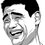# A different DISCUSSION ?

Hey today i will try to introduce the subject i like the least BIOLOGY ! ( i hope it would be appreciated ...:D) Actually i found a good problem that i would like to share at my level so you can think of it as a mathematical problem and solve it that way if you could ! (typical biology problem) the problem as told by my friend: 16% of a population have blood group "O" and 25% of the population have blood group pure "A". Now from the above data compute what %(percent) of population will have blood group "AB" ?

If it's not appropriate to post these here please tell me !Note by Ritvik Choudhary
6 years, 12 months ago

This discussion board is a place to discuss our Daily Challenges and the math and science related to those challenges. Explanations are more than just a solution — they should explain the steps and thinking strategies that you used to obtain the solution. Comments should further the discussion of math and science.

When posting on Brilliant:

• Use the emojis to react to an explanation, whether you're congratulating a job well done , or just really confused .
• Ask specific questions about the challenge or the steps in somebody's explanation. Well-posed questions can add a lot to the discussion, but posting "I don't understand!" doesn't help anyone.
• Try to contribute something new to the discussion, whether it is an extension, generalization or other idea related to the challenge.

MarkdownAppears as
*italics* or _italics_ italics
**bold** or __bold__ bold
- bulleted- list
• bulleted
• list
1. numbered2. list
1. numbered
2. list
Note: you must add a full line of space before and after lists for them to show up correctly
paragraph 1paragraph 2

paragraph 1

paragraph 2

[example link](https://brilliant.org)example link
> This is a quote
This is a quote
    # I indented these lines
# 4 spaces, and now they show
# up as a code block.

print "hello world"
# I indented these lines
# 4 spaces, and now they show
# up as a code block.

print "hello world"
MathAppears as
Remember to wrap math in $$ ... $$ or $ ... $ to ensure proper formatting.
2 \times 3 $2 \times 3$
2^{34} $2^{34}$
a_{i-1} $a_{i-1}$
\frac{2}{3} $\frac{2}{3}$
\sqrt{2} $\sqrt{2}$
\sum_{i=1}^3 $\sum_{i=1}^3$
\sin \theta $\sin \theta$
\boxed{123} $\boxed{123}$

Sort by:

Using the Hardy-Weinberg equations for three alleles, we get: $A+B+O=1$ $A^2+B^2+O^2+2AB+2AO+2BO=1$ We also know: $O^2=.16$ $A^2=.25$ Which implies: $O=.4$ $A=.5$ Thus $B=.1$ and the frequency of blood group AB is $2AB=.1$ Perhaps Brilliant is not yet the place for such questions. These kinds of topics require an odd background to solve.

- 6 years, 11 months ago

Shouldn't that be $A^2 + B^2 + O^2 + 2AB + 2AO + 2BO = 1$?

- 6 years, 11 months ago

Thanks.

- 6 years, 11 months ago

Shall we assume Hardy-Weinberg Equilibrium?

- 6 years, 12 months ago

Yeah of course you can if by this you can get to the answer you can !!

- 6 years, 12 months ago